# Non-ideal relay

The hysteresis non-linearity denoted by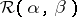, with thresholdsand, and defined for a continuous input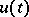,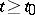, and an initial state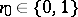by the formulas (see Fig.a1.)where, that is,denotes the last switching moment. The input–output operators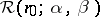are discontinuous in the usual function spaces. These operators are monotone in a natural sense, which allows one to use the powerful methods of the theory of semi-ordered spaces in the analysis of systems with non-ideal relays.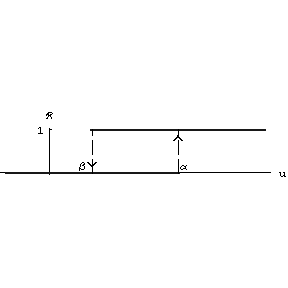Figure: n110060a

Non-ideal relay

The Preisach–Giltay model of ferromagnetic hysteresis is described as the spectral decomposition in a continual system of non-ideal relays in the following way. Let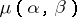be a finite Borel measure in the half-plane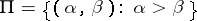. The input–output operators of the Preisach–Giltay hysteresis non-linearity at a given continuous input,, and initial stateis defined by the formula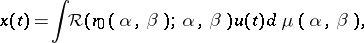where the measurable function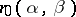describes the internal state of the non-linearity at the initial moment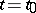. In contrast to the individual non-ideal relay, the operators of a Preisach–Giltay non-linearity are continuous in the space of continuous functions, provided that the measureis absolutely continuous with respect to the Lebesgue measure (cf. Absolute continuity). For detailed properties of Preisach–Giltay hysteresis and further generalizations see [a1], [a2] and the references therein.